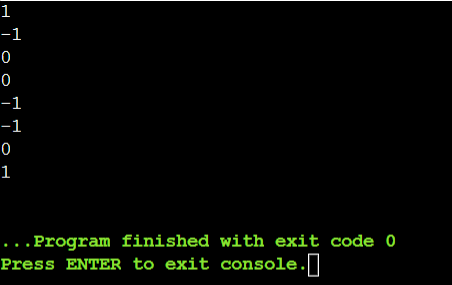# Family of functions (template function) in C++

## Question

Define a family of functions (a templated function) named occurrences that determine whether an array of any type and specified length contains a value specified as a parameter. This function returns:

0  if the value is found exactly once in the array

1 if the value is found multiple times in the array

Specialize the function for the std::string type to count all elements of the array that contain as a substring the value. For example, in the array( “abcd”, “bcde” } the string “ab” appears once (return 0), the string “cd” appears twice (return 1), and the string “ef” doesn’t appear at all (return -1).

Assume all parameters are valid

A type that uses this template must include in its definition certain functions and/or operators. Identify each function and/or operator that your template assumes is defined. You may do so in the form of an exact prototype or an English descriptive phrase.

## Summary

In this question, we will use the concept of function overloading. We will create a template function in C++. Here, we have defined three functions. First for getting the frequency of an element in an integer array. Second for getting the frequency of an element in a short array and third for getting the frequency in a string array.

If the searching element is not present in the given array, it returns -1, if it is present one time then it returns 0, and if it is present multiple times then it returns 1.

## Explanation

To check the occurrences of a given element, we will use three different functions for different types of data types. We will define one variable to count the frequencies of the given element and we will use a loop to go through every element in the array. So for three different functions, we will create a template function in C++.

## Code

#include <iostream>
using namespace std;
/* function to find the number of frequency of an integer in
an integer array */
int frequency(int a[],int n,int key){
int c=0;
for(int i=0;i<n;i++){
if(a[i]==key)
c++;
}
/* if no frequency */
if(c==0)
return -1;
/* if one frequency */
else if(c==1)
return 0;
/* if multiple frequency */
else if(c>1)
return 1;
}
/* function to find the number of frequency of an integer in
a short array */
int frequency(short a[],int n,int key){
int c=0;
for(int i=0;i<n;i++){
if(a[i]==key)
c++;
}
/* if no frequency */
if(c==0)
return -1;
/* if one frequency */
else if(c==1)
return 0;
/* if multiple frequency */
else if(c>1)
return 1;
}
/* function to find the number of frequencies of a string in
a string array */
int frequency(string a[],int n,string key){
int c=0;
for(int i=0;i<n;i++){
if(a[i].find(key)!=-1)
c++;
}
/* if no frequency */
if(c==0)
return -1;
/* if one frequency */
else if(c==1)
return 0;
/* if multiple frequency */
else if(c>1)
return 1;
}
/* driver code */
int main()
{
{
int a[]{ 6, 3, 8, 7, 3, 2, 3 };
// number 4 appears in two consecutive locations
cout << frequency(a, 7u, 3) << "\n"; // prints 1
cout << frequency(a, 7u, 9) << "\n"; // prints -1
cout << frequency(a, 7u, 8) << "\n"; // prints 0
}

{
short a[]{ 1, 2, 3, 4 };
cout << frequency(a, 4u, 2) << "\n"; // prints 0
cout << frequency(a, 4u, 5) << "\n"; // prints -1
}

{
string a[]{ "abcd", "bcde", "cdef" };
cout << frequency(a, 1u, "de") << "\n"; // prints -1
cout << frequency(a, 2u, "de") << "\n"; // prints 0
cout << frequency(a, 3u, "de") << "\n"; // prints 1
}
}

## Output